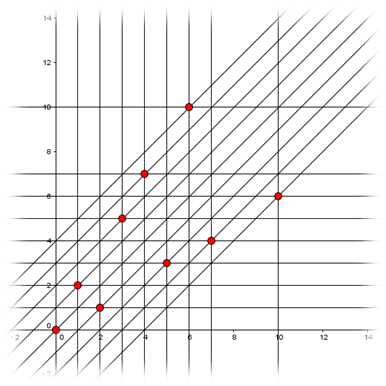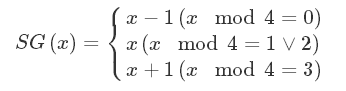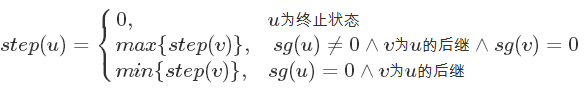# 博弈论入门：

## 尼姆博弈(Nim)：

### EX：nimk游戏

1、终止局面为先手必败(显然)

2、任意先手必胜的局面都能传变成先手必败的局面。

3、任意先手必败的局面都不能转变为先手必胜的局面。

## 威佐夫博弈：

$(0,0),(1,2),(3,5),(4,7),(6,10)……$我们可以将两堆石子看成是棋盘上一个点的横纵坐标，那么游戏就变成了：棋盘上有一个点，每次每人只能将棋子往左或往下移动任意个格子或往左下移动任意个格子，不能移动的人输。终止节点显然是$(0,0)$，我们将能一步到达$(0,0)$的点都染色，那么这些点就都是必胜态，再找到横纵坐标之和最小的没被染色的点，这个点就是下一个必败态，由此就能画出上图。

# 组合游戏：

1、两个人进行博弈

2、两人轮流进行决策且做出的决策都对自己有利

3、游戏中同一个局势不能多次到达且游戏不能有平局

4、当有人无法做出决策时游戏结束，无法做出决策的人输

5、游戏可以在优先步数内结束

6、任意游戏者在某一局势时能做出决策的集合只与当前局势有关，与游戏者本身无关

## 必胜态与必败态：

$P-position$：必败态(简称$P$)，即在决策前就处于这种状态的人必败

$N-position$：必胜态(简称$N$)，即在决策前就处于这种状态的人必胜

1、无法移动的状态为$P$

2、可以移动到$P$的状态为$N$

3、任意移动都会到$N$的状态为$P$

## SG函数及SG定理

$SG$函数是人们在研究博弈问题上迈出的一大步，人们不再一味的通过找规律来得到博弈结论，而是将博弈问题的每一个状态进行量化分析并总结出了某些普遍性的规律。

$SG$函数描述的是博弈中某个状态量化后的值，$SG(x)=mex\{SG(y)|x\ can\ go\ to\ y\}$

1、终止状态$SG$值为$0$，因为它没有出边(即可到达的状态)

2、$SG$值为$0$的状态为必败态，它的后继状态$SG$值都不为$0$，满足必败态定义

3、SG值不为$0$的状态为必胜态，它的后继状态$SG$值有为$0$的状态，满足必胜态定义

## anti-SG与SJ定理

$anti-SG$组合游戏的其他规则与$SG$组合游戏规则相同，但它规定决策集合为空者获胜。

1、每堆石子数异或和不为$0$且至少有一堆石子数大于$1$

2、每堆石子数异或和为$0$且每堆石子只有$1$个

## multi-SG

$multi-SG$游戏的规则与$SG$游戏相同但多出一个可以将单一游戏分成多个单一游戏的操作。对于分裂单一游戏的操作，分裂后的状态的$SG$值可以看做是分裂出的单一游戏$SG$值的异或和。

$sg(1)=mex\{sg(0)\}$

$sg(2)=mex\{sg(0),sg(1)\}=2$

$sg(3)=mex\{sg(0),sg(1),sg(2),sg(1,2)\}=4$

$sg(4)=mex\{sg(0),sg(1),sg(2),sg(1,3),sg(2,2),sg(3)\}=3$## every-SG

$every-SG$游戏规定对于任意未结束的单一游戏，游戏者必须对它进行决策，游戏结束时间决定于最后一个结束的单一游戏，胜负则取决于最后一个结束的单一游戏的输赢。### 对于every-SG游戏先手必胜当且仅当单一游戏step最大的一个为奇数。

posted @ 2018-09-22 16:35  The_Virtuoso  阅读(5649)  评论(4编辑  收藏  举报